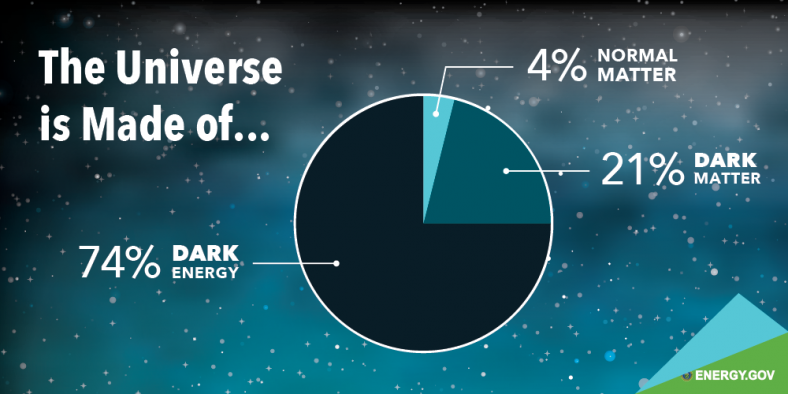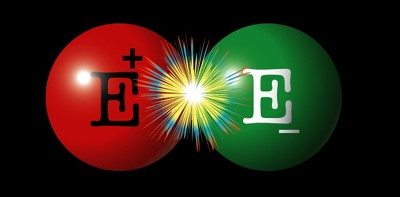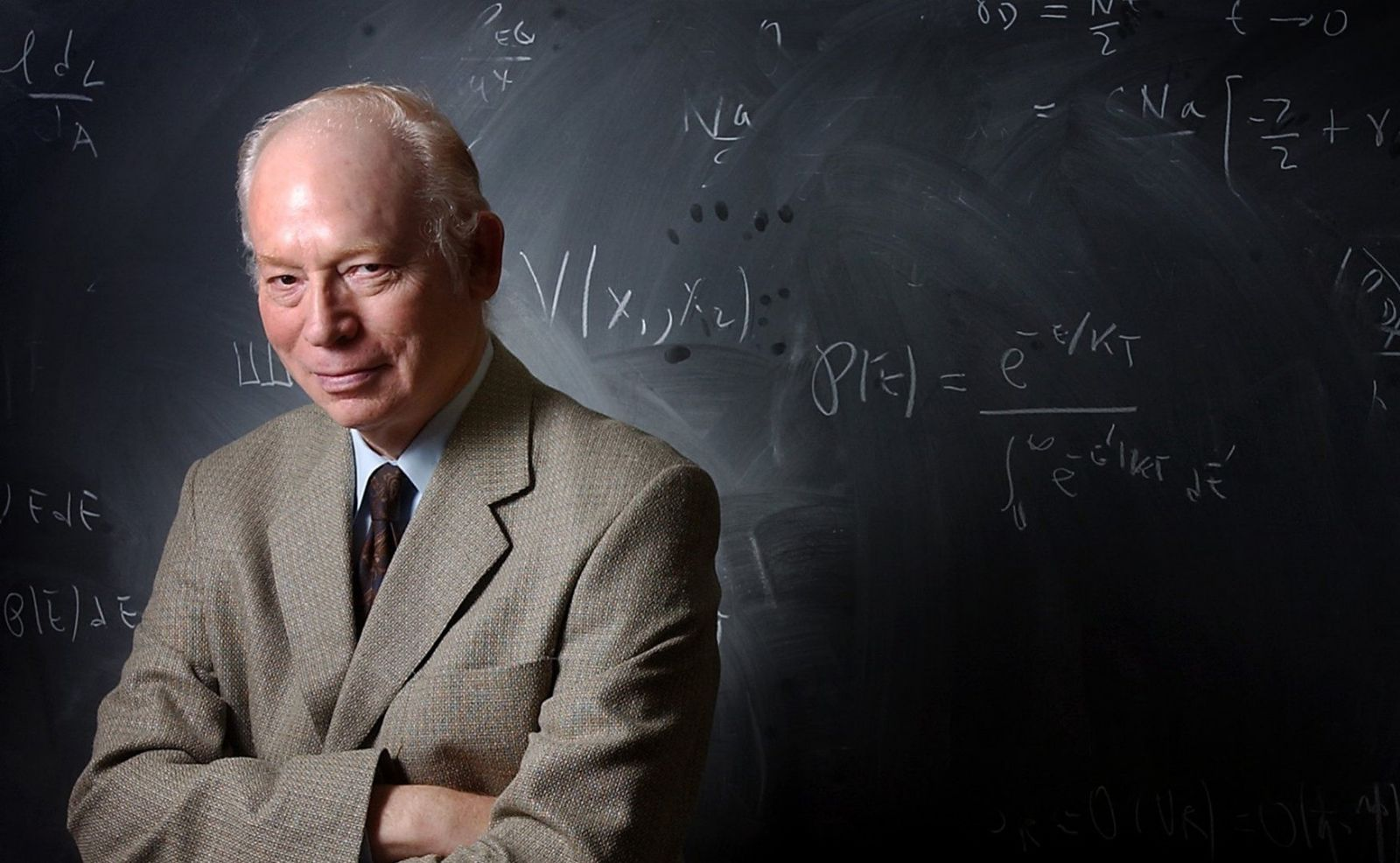# Problems in the standard model: a journey beyond it!The model which describes the world around us is called “The Standard Model” of particle physics. It includes many particles like the very well know electron, the photon, the Higgs particle (sometimes called The God Particle), the quarks and many more.

#### June 5, 2018 by andrea0292

With the help of the standard model we can explain many things which happen in nature, for example, we can understand:

- why and how particles get a mass,

- why we daily interact with photons (light) but we cannot notice the tons of neutrinos which pass through our bodies every single second,

- why the sun burns

- etcetera

Some of the most astonishing scientific measurements in the world are predicted with extreme accuracy within the framework of this model. Nevertheless, there are a lot of things in our universe that The Standard Model cannot explain, and I would say that this is super fascinating. It means that there is still work to do, things to understand and stimulating open questions some of which I will try to describe in the following.

# Dark Matter

### Galaxies speeding up

When we look at galaxies in our universe, we notice something really peculiar: they are rotating too fast.

The rotational velocity of a star in a galaxy is determined, following Newton laws, by the mass enclosed in the region between the star itself and the center of the observed galaxy. We infer this mass from astronomical observation, using electromagnetic interactions.

Matter in stars and galaxies absorb, reflect or emit light that we are able to detect letting us to do estimations of the mass in the observed region. However, if we use this value to compute the rotational velocity of stars with our current theory of gravitation, we get a result which does not agree with data.

Data in fact seem to tell us that there is extra matter in those regions, and actually that this matter should be much more abundant than the matter we observe.

This new kind of “unknown” matter is the so called Dark Matter.

### Why Dark?

First of all because, as stated above, it doesn’t interact with the electromagnetic force, otherwise we should have seen it (as we see a normal star that emits its own light or the planets that simply reflect it) and there seems not to be a particle of the Standard Model with the required properties to be a candidate for this new kind of matter.

The only thing we know for sure is that Dark Matter has to interact gravitationally (to modify the rotational speeds of galaxies through its mass) and weakly enough in order to be so difficult to detect.

Moreover, it turns out that apparently only 4% of our universe is made of the matter we know and “see”, which we call “baryonic matter” (the stuff of which atoms are made); other 21% of the universe is made of this unknown dark matter and the rest is what is called dark energy (that will be explained in what follows). Essentially, we just understand around 4% of our universe; isn’t it fascinating?

# Dark Energy

We have just said that 4% of our universe is made of baryonic matter and other 21% of it by Dark matter; so what is that makes up the remaining 74% of our universe? It is the so called Dark Energy.

In 1998 astronomers found, observing the exploding stars in different galaxies, that the expansion of the universe is speeding up!

The fact that the universe is expanding was known decades ago, but physicists always thought that the expansion was decelerating; in fact, using the laws of general relativity, and even just the common sense derived from Newtonian Gravitation, one expects that the mass enclosed in the universe slows down the expansion, through gravitational attraction.

The observation that the expansion of the universe was accelerating was like looking at a bullet which comes back once discharged.

If we accept the validity of the general theory of relativity, this can only be explained by an energy that is not contained within standard particle masses, but it is instead inherent to space itself.

This “Dark Energy” produces a sort of antigravity which pushes the galaxies apart. Summing up, we live in an expanding universe where most of the mass and energy (equivalent concepts in the realm of relativistic theories) comes from unknown forms of dark energy and dark matter. Only a small part of this “Dark Universe” consists of the standard baryonic matter which is the fabric of stars, planets and, of course, us.Fig.1 Resume of the content of the universe we live in. Most of it is made of things we do not understand.

# Neutrino masses

### Massive or not massive, that is the question

In the Standard Model neutrinos are massless particles which have no electric charge and interact very weakly.

Neutrinos exist in three different flavors: electron, muon and tau neutrinos. They were first proposed by Pauli in 1930 while investigating the conundrum of radioactive beta decay. The famous scientist wrote in a letter to a colleague “I have done something very bad today by proposing a particle that cannot be detected; it is something no theorist should ever do”.

As you see, even the smartest scientists make mistakes; in fact, neutrinos were indeed discovered and since then they have become a fundamental piece of the Standard Model puzzle!.

However, the Standard Model neutrinos do not have a mass, while current experiments have shown that they actually DO have a mass; it is really tiny, more than a million times smaller than electron mass, but it is very existence gives rise to important physical phenomena like neutrino oscillations.

### Neutrino as a dark matter candidate?

At this point the careful reader could stop and think: “Wait a moment, neutrino have a mass, so they interact gravitationally; they don’t have an electrical charge, so they do NOT interact with electromagnetic force, and they interact weakly, with no strong forces into the game. Could neutrinos be the source of dark matter we are looking for?

Well done, this is not a crazy idea at all. Actually, I lied a bit in the “Dark Matter” section when I said that among the particles of the Standard Model none of them has the expected properties to become a dark matter candidate. Neutrinos have most of the properties to convert them into a good candidate and, in fact, many physicists have proposed them as the main source for dark matter.

Unfortunately, their mass seems to be insufficient and moreover we could get conflicting predictions regarding galactic structures. In fact, the lighter is the dark matter particle the more relativistic it will be, leading to small inhomogeneities on galaxy-scale.

### Why so small?

But why neutrino masses are so small? This is another open question in particle physics which we would like to address; there are many proposed solutions in the scientific literature, but the most appealing and generally accepted one is that there is a kind of new very massive particles, called sterile neutrinos.

These sterile neutrinos are even more elusive than “normal” or active neutrinos (light neutrinos are also called active), because they do not interact neither through weak interactions. However, with a mechanism called “seesaw” it is possible to explain the tiny mass of active neutrinos: the larger is the mass of sterile neutrinos, the smaller will be the one of the active. Therefore very massive (therefore very difficult to produce at colliders) sterile neutrinos imply very light active neutrinos.Fig.2 Masses of particles in the Standard Model. The neutrinos have masses much smaller than all the other particles. Why so small?

# Matter Antimatter Asymmetry

### Dr. Jekyll and Mr.Hyde

In the study of particle physics a fundamental equation which describes the rules of nature is the Dirac Equation; this equation tells us that for every particle in nature there is a corresponding anti-particle, which has the same mass, spin, type of interactions, but opposite charge.

It is somehow the particle equivalent to the algebraic equation x2=1, which has the two solutions ±1.

The anti-particle of the electron, for example, is called positron, the one of the muon, anti-muon, the one of the tau anti-tau and so on. When a particle and an anti-particle meet they annihilate each other emitting energy and disappearing. Therefore, it is intuitive to understand that if there is in the universe the same number of particles and anti-particles they would have with time annihilated each other and we wouldn’t be here.

Fortunately for us, it turns out that in the universe there seems to be many more particles than anti-particles; for example, looking at cosmic rays we found evidence of protons and anti-protons, but anti-protons constitute only 1%.

### Baryogenesis

This particle-antiparticle asymmetry cannot be explained with the Standard Model. One, in fact, would expect that at the beginning of everything, after the big bang, particles and anti-particles were equally distributed and after that some kind of dynamical mechanism would have generated the asymmetry we observe today. We call this dynamical mechanism Baryogenesis.

There are different models for Baryogenesis. One of them involves the decay or the oscillations of the sterile neutrinos discussed above. None of them is definitive, and all of them involve physics beyond the standard model: new particles, new interactions.

### Think like a physicist

One could argue that it would be enough just to postulate this asymmetry since the beginning, immediately after the Big Bang; in fact, there is nothing wrong with this, but this is not the way physicists like to think. We don’t like coincidences, we don’t like extremely fine-tuned conditions; in principle we think we should be able to explain everything in a rigorous, consistent and unified way.Fig.3 An electron and a positron annihilate and produce energy. If the Universe was composed by the same amount of matter and antimatter, we would not be here now.

# STRONG CP PROBLEM

### SYMMETRIES

A fundamental ingredient to build the Standard Model and understand the world we live in is the concept of Symmetry.

In everyday life we experience many symmetries, most of them related to objects; a cube is a perfect example: every face, every edge, every corner is the same and if we rotate the cube in certain ways its appearance does not change (a sphere is invariant with respect any possible rotation).

A symmetry is essentially a principle of invariance and physics is intrinsically related to symmetries, not so much symmetries of objects but mainly symmetries of laws.

Roughly speaking, a physics law is said to respect a given symmetry if it doesn’t change if we change the point of view of the observer. For instance, Newton laws of gravitation stay the same if we rotate our laboratory (rotational symmetry) or if we change the reference point we use to measure distances (translational symmetry).

### Mirror Symmetry and CP

The mirror symmetry states that physical laws do not change if we observe them in a mirror, and it was generally taken for granted that physics respected this symmetry.

However, in 1957 experiments showed with no doubts that weak nuclear force does not obey mirror symmetry. More technically this symmetry is called Parity transformation, and together with the Charge conjugation, that is the exchange of particle and anti-particle, they form the important combination CP. This is a very fundamental symmetry in which is almost always respected; almost because again we observe small violation of this symmetry in weak interactions.

A violation of CP coincides with a violation of time reversal. In fact, there is an important theorem in Theoretical Physics which says that the symmetry CPT, that is to say the combination of CP and time reversal, must be conserved. Therefore, observing a violation of CP coincides with an observation of violation of time reversal and vice versa.

### CP STRONG

Usually we build a theory respecting a given symmetry if we experimentally observe that nature preserves that symmetry; however, we just said that CP is not respected by Weak Interactions, therefore there is no reason why we should impose this symmetry in the other sectors of the standard model, like the sector of strong interactions.

However, no violation of CP-symmetry is observed regarding strong interactions. Why don’t we like this? Once again, we physicists don’t like coincidences or fine tuning.

In fact, experimentally we measure a tiny value for the electric dipole moment for the neutron. This quantity is directly related to a parameter α which parameterizes the violation of CP in the strong interactions: a small α implies a small violation of CP in strong interactions.

It turns out that the measured electric dipole moment is explained only if α is extremely small, roughly α~10-10. Why α is so small and CP seems to be conserved in Strong Interaction? Is there any dynamical mechanism (like in the case of matter antimatter asymmetry) which leads to this super tiny value? This is the Strong CP problem.

# Quantum gravity

### A theory of everything

If one opens a book of Quantum Field Theory (QFT), that is the subject that explains the content of matter and the interactions of the Standard Model, will notice that there is space for the discussion of three types of interaction: the electromagnetic, the weak and strong forces, which can be unified together in a beautiful theory.

In particular, QFT arises from the fusion of quantum mechanics (QM) and special relativity, but in all this picture there is one, very common, force which doesn’t fit: gravity, or more precisely our current theory of gravity, i.e. Einstein’s general relativity (GR).

Up to now all attempts to search for a synthesis of QM and GR have failed, even if scientist of the category of Einstein, Hawking or Witten have tried really hard. The quantization of GR is expected to lead to the “theory of everything”, the dream of all physicists: a theory which englobes all the forces known in nature in a uniform and elegant model.

Of course, these are just a few of the open questions we currently have in physics and I think all scientifically minded students have the ambition to find the answer for at least one of them. The Standard Model is incomplete and for sure it needs to be “completed”; or perhaps we need a completely new theory, which will be able to reproduce the excellent results of the standard model and improve it, as the General Relativity did with Newtonian Gravity.

One of the main builder of the Standard Model, Nobel prize laureate Steven Weinberg, says: “I still hold the hope that one day a paper posted in the arXiv preprint server by some previously unknown graduate student will turn the SM on its head – a 21st century model of particles that incorporates dark matter and dark energy and has all the hallmarks of being a correct theory, using ideas no one had thought of before”.Fig. 4 Nobel Prize-winning Steven Weinberg, one of the most brilliant physicist of last century.